# Current

Letbe an-dimensional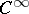-manifold with countable basis (cf. also Differentiable manifold) and let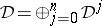, wheredenotes the vector space of compactly supported differential forms of degreeon(cf. also Differential form). Endow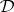with the usual structure of a Fréchet space by declaring thattends toif there exists a compact set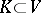such that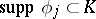for alland the coefficients of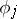and all their derivatives tend uniformly to those of.

A current onis an element of the dual space. The idea of currents was introduced by G. de Rham in [a6], to obtain a homology theory including both forms and chains, but a precise definition, see [a7], [a8], became only possible after distributions (cf. also Generalized function) had been introduced by L. Schwartz. See also (the editorial comments to) Differential form, whose notation is used here too.

While exterior products of currents are in general undefined, exterior differentiation can be defined by duality. If the action of a currentof degreeon a formis denoted by, then one defines the exterior differentialby. In particular, the notions of closed and exact currents are defined.

Now, letbe a complex manifold. One has the splitting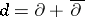for currents just as for forms.

A theorem of P. Lelong [a4] states that any pure-dimensional analytic subsetof a Hermitian complex manifold has locally finite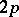-volume. As a consequence one can define the current of integration overby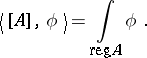Here, the integration is over the regular points of(cf. also Analytic set).is a-closed current of bi-dimension. Moreover,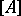is positive, that is,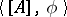is positive for forms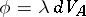, withandbeing the volume form on the regular points of. See also [a2], [a5].

Thus, currents can be viewed as an extension of the notion of analytic manifold. This idea has been very fruitful in complex analysis. See e.g. [a1], [a3] and their references.